Ellipse - Defination

Chapter 10 Class 11 Conic Sections
Concept wise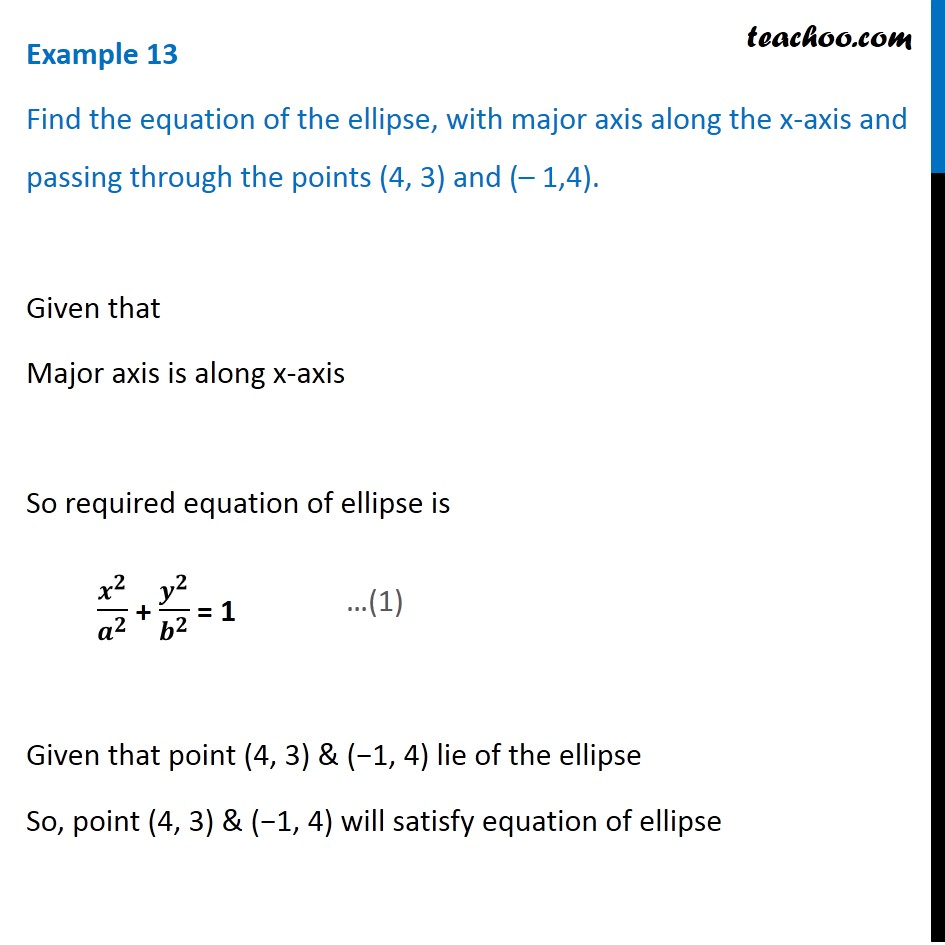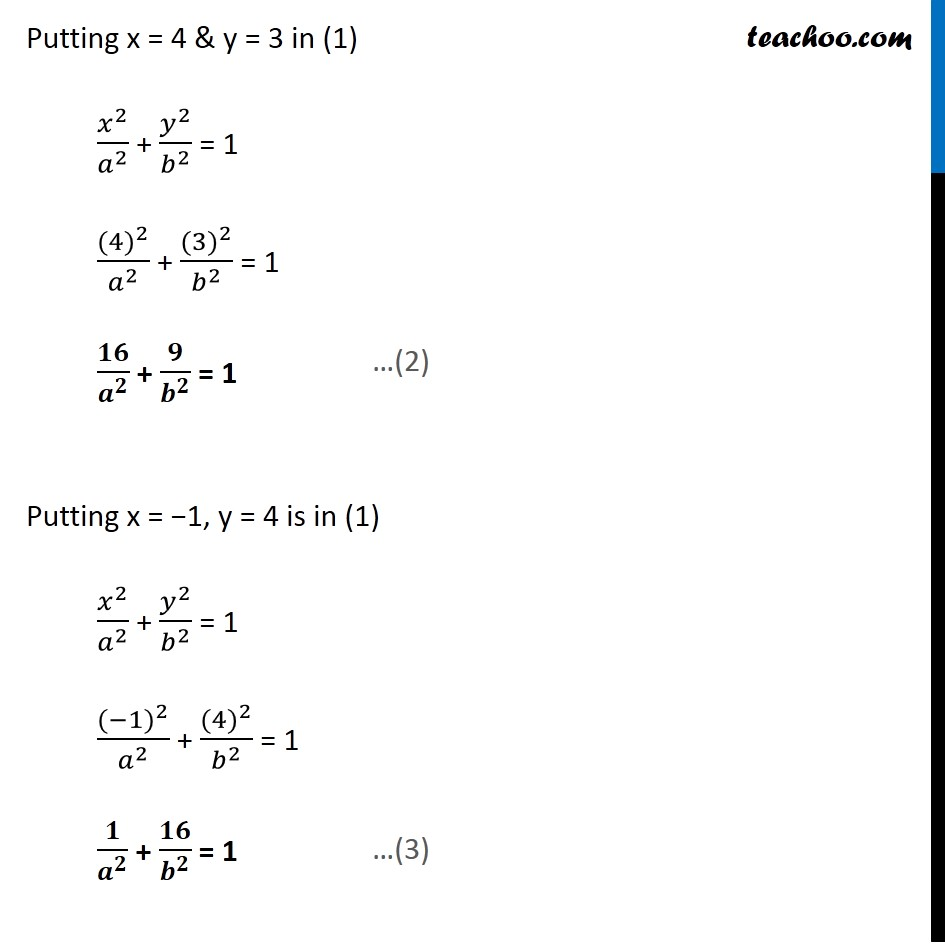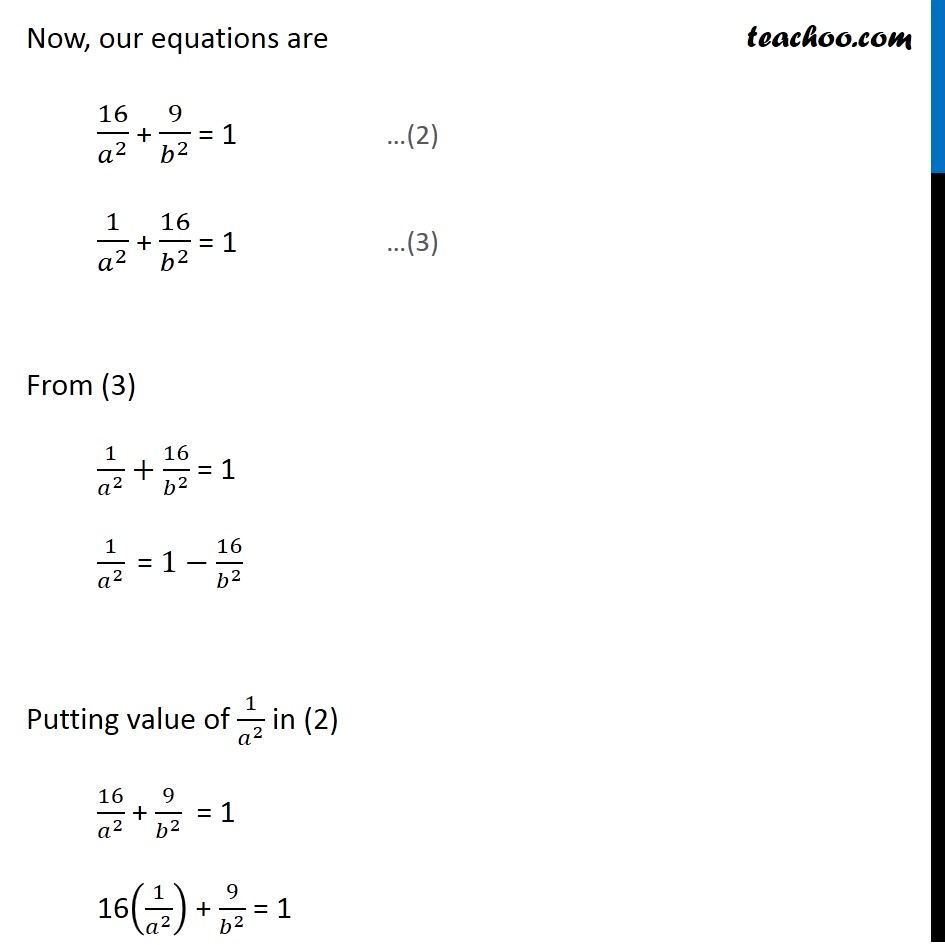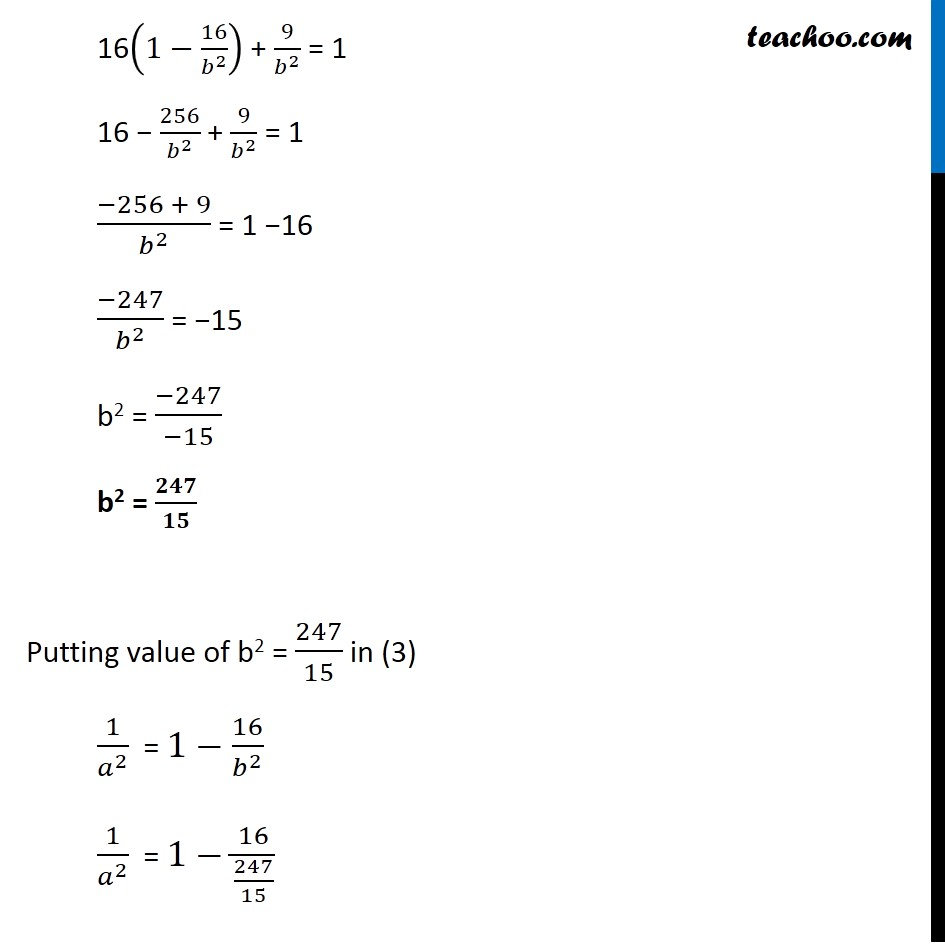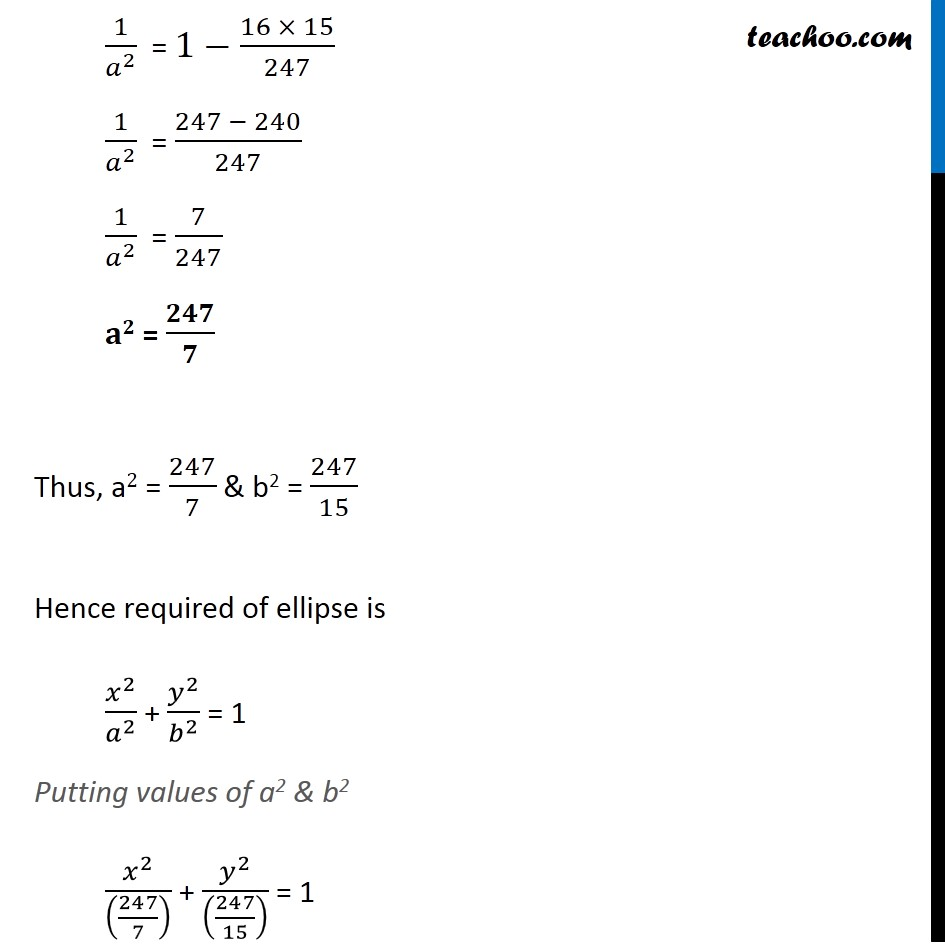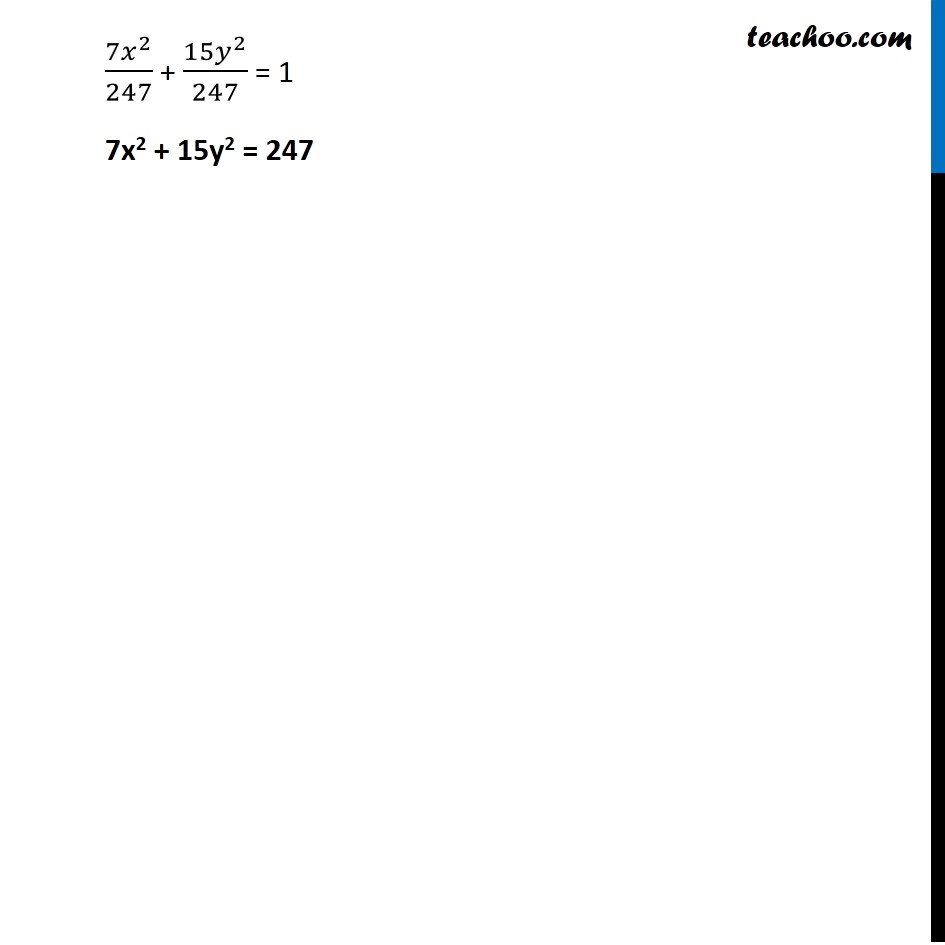Learn in your speed, with individual attention - Teachoo Maths 1-on-1 Class

### Transcript

Example 13 Find the equation of the ellipse, with major axis along the x-axis and passing through the points (4, 3) and (– 1,4). Given that Major axis is along x-axis So required equation of ellipse is 𝒙^𝟐/𝒂^𝟐 + 𝒚^𝟐/𝒃^𝟐 = 1 Given that point (4, 3) & (−1, 4) lie of the ellipse So, point (4, 3) & (−1, 4) will satisfy equation of ellipse Putting x = 4 & y = 3 in (1) 𝑥^2/𝑎^2 + 𝑦^2/𝑏^2 = 1 〖(4)〗^2/𝑎^2 + 〖(3)〗^2/𝑏^2 = 1 𝟏𝟔/𝒂^𝟐 + 𝟗/𝒃^𝟐 = 1 Putting x = −1, y = 4 is in (1) 𝑥^2/𝑎^2 + 𝑦^2/𝑏^2 = 1 〖(−1)〗^2/𝑎^2 + 〖(4)〗^2/𝑏^2 = 1 𝟏/𝒂^𝟐 + 𝟏𝟔/𝒃^𝟐 = 1 Now, our equations are 16/𝑎^2 + 9/𝑏^2 = 1 1/𝑎^2 + 16/𝑏^2 = 1 From (3) 1/𝑎^2 +16/𝑏^2 = 1 1/𝑎^2 " "= 1−16/𝑏^2 Putting value of 1/𝑎^2 in (2) 16/𝑎^2 + 9/𝑏^2 = 1 16(1/𝑎^2 ) + 9/𝑏^2 = 1 16(1−16/𝑏^2 ) + 9/𝑏^2 = 1 16 − 256/𝑏^2 + 9/𝑏^2 = 1 (−256 + 9)/𝑏^2 = 1 −16 (−247)/𝑏^2 = −15 b2 = (−247)/(−15) b2 = 𝟐𝟒𝟕/𝟏𝟓 Putting value of b2 = 247/15 in (3) 1/𝑎^2 " "= 1−16/𝑏^2 1/𝑎^2 " "= 1−16/(247/15) 1/𝑎^2 " "= 1−(16 × 15)/247 1/𝑎^2 " "= (247 − 240)/247 1/𝑎^2 " "= 7/247 𝐚𝟐 = 𝟐𝟒𝟕/𝟕 Thus, a2 = 247/7 & b2 = 247/15 Hence required of ellipse is 𝑥^2/𝑎^2 + 𝑦^2/𝑏^2 = 1 Putting values of a2 & b2 𝑥^2/((247/7) ) + 𝑦^2/((247/15) ) = 1 1/𝑎^2 " "= 1−(16 × 15)/247 1/𝑎^2 " "= (247 − 240)/247 1/𝑎^2 " "= 7/247 𝐚𝟐 = 𝟐𝟒𝟕/𝟕 Thus, a2 = 247/7 & b2 = 247/15 Hence required of ellipse is 𝑥^2/𝑎^2 + 𝑦^2/𝑏^2 = 1 Putting values of a2 & b2 𝑥^2/((247/7) ) + 𝑦^2/((247/15) ) = 1 (7𝑥^2)/247 + (15𝑦^2)/247 = 1 7x2 + 15y2 = 247